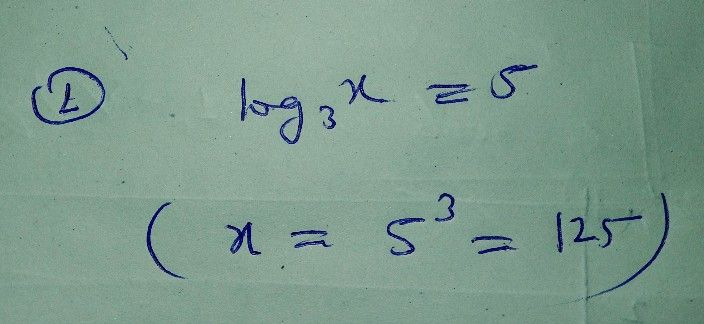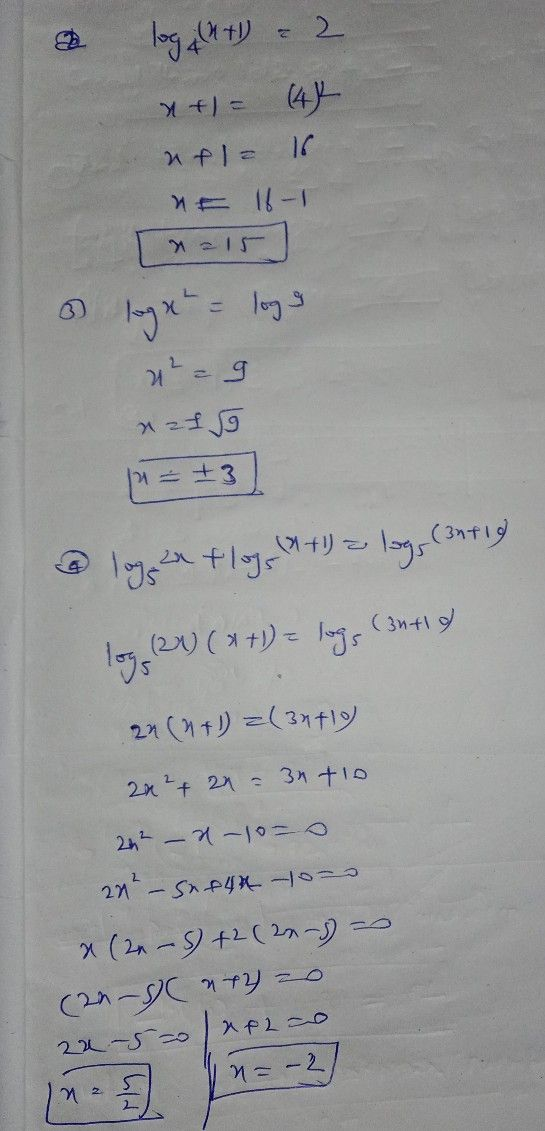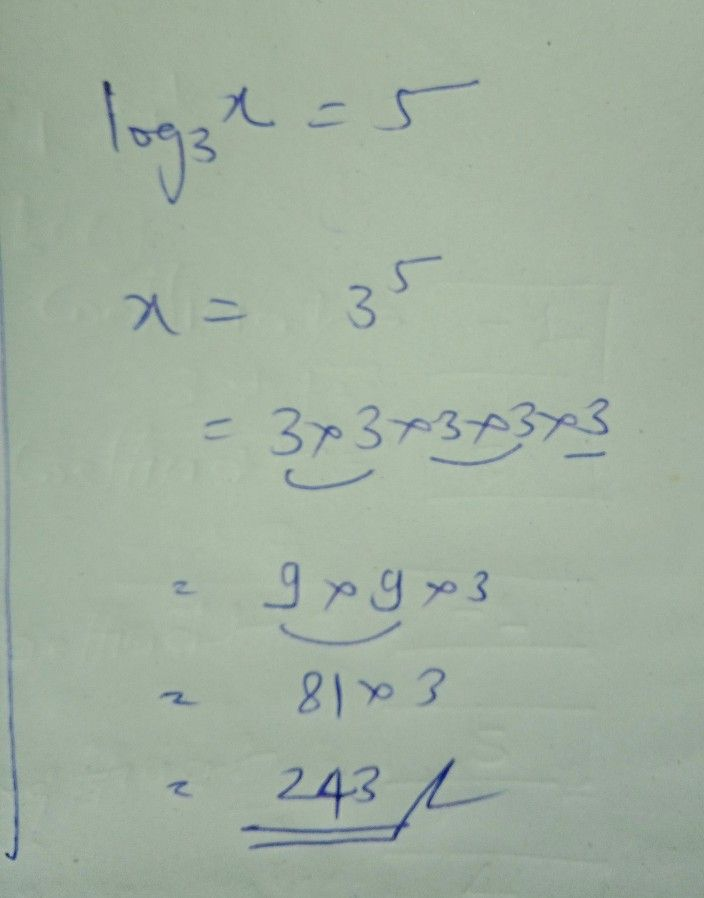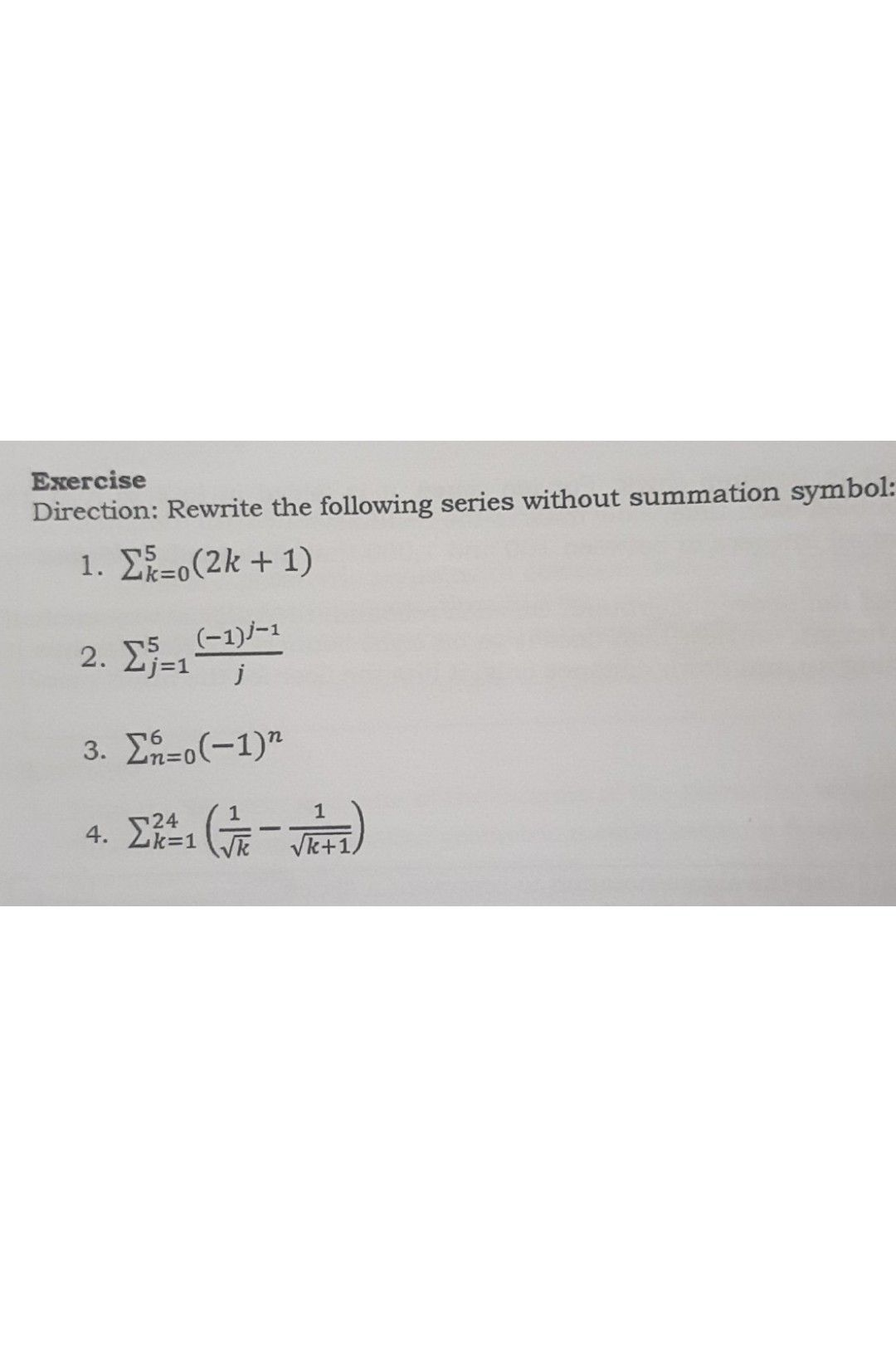Symbol
ProblemSolve the following logarithmic equations. $1.$ $log _{3}x=5$ $2$ $log4\left(x+1\right)$ $=2$ $3$ $lnx^{2}=ln9$ $4$ $log5^{2x+log _{5}\left(x+1\right)}=log5\left(3x+10\right)$
Other
Search count: 116
SolutionQanda teacher - ManojKumarStudent
ok?
thank youQanda teacher - ManojKumarI really sorry for first picture
but second is absolutely correctStudentQanda teacher - ManojKumar
okStudent
do you have other solution sir for number 1?
thank you teacher?Godbless youQanda teacher - ManojKumar
it is formula based question